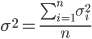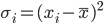# thesIt

•#### Arif 03:07:29 pm on August 24, 2010 | 0 | # | Tags: error, Geman 1992, MSE, neural network, poor data, Silvert 1998, variance

Bias-variance dilemma (Geman et al., 1992). It can be demonstrated that the mean square value of the estimation error between the function to be modelled and the neural network consists of the sum of the (squared) bias and variance. With a neural network using a training set of fixed size, a small bias can only be achieved with a large variance (Haykin, 1994). This dilemma can be circumvented if the training set is made very large, but if the total amount of data is limited, this may not be possible.

• #### Arif 10:27:38 pm on June 28, 2010 | 0 | # | Tags: basic, standard deviation, statistics, variance

Easiest description for standard deviation definition is distance from mean (expected value) as shown in this graphical depictionwhere all the values fall at σ distance within the dotted circle radius. Of course a more real-life situation is shown aswhere σ is the square root of the following mean$\sigma^2 =\frac{\sum_{i=1}^{n}{\sigma_{i}^2}}{n}$

σ² a.k.a. variance is averaged quadratic distances. Explanation:

Distance may have several concepts, in this variance description, distance shows “how far” a value is from its population expected value (mean). Quadratic form of this “how far” is$\sigma_i=(x_i-\bar{x} )^2$